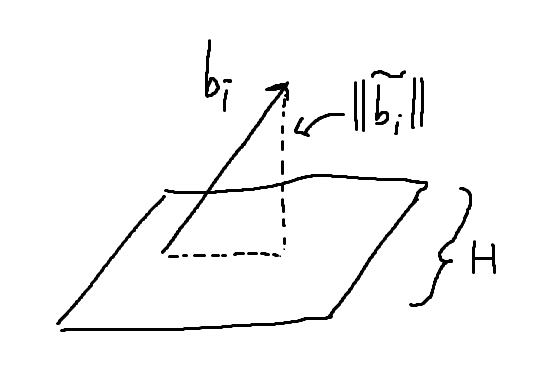# Proof of the inequality of a reduced basis

• A
• Peter_Newman
In summary, the LLL-reduced basis satisfies the following property (Reference): For ##1 \leq i \leq n## concider ##H = span(b_1,...,b_{i-1},b_{i+1},...,b_n)##. Show that ##2^{-n(n-1)/4}||b_i|| \leq dist(H,b_i) \leq || b_i ||##. Hint use ##\prod ||b_i|| \leq 2^{n(n-1)/4} det(\Lambda)##.

#### Peter_Newman

TL;DR Summary
For ##1 \leq i \leq n## concider ##H = span(b_1,...,b_{i-1},b_{i+1},...,b_n)##. Show that ##2^{-n(n-1)/4}||b_i|| \leq dist(H,b_i) \leq || b_i ||##. Hint use ##\prod ||b_i|| \leq 2^{n(n-1)/4} det(\Lambda)##. Own reasoning below.

I would like to show that a LLL-reduced basis satisfies the following property (Reference):

For ##1 \leq i \leq n## concider ##H = span(b_1,...,b_{i-1},b_{i+1},...,b_n)##. Show that ##2^{-n(n-1)/4}||b_i|| \leq dist(H,b_i) \leq || b_i ||##. Hint use ##\prod ||b_i|| \leq 2^{n(n-1)/4} det(\Lambda)##.

My Idea:
I also have a first approach for the part ##dist(H,b_i) \leq || b_i ||## of the inequality, which I want to present here based on a picture, which is used to explain my thought:So based on the picture we can see that the norm of the orthogonal part (##\tilde{b_i}##) of the vector ##b_i## gives the distance. Therefor I would argue that ##dist(H,b_i) = ||\tilde{b_i}||## and further ##||\tilde{b_i}|| \leq ||b_i||## since the norm of a Gram-Schmidt vector is less than the norm of the regular vector. If I put this together it makes for me a valid argument to say ##dist(H,b_i) \leq || b_i ||##. This would prove one part of the inequality.

Problem:
However I do not manage to show ##2^{-n(n-1)/4}||b_i|| \leq dist(H,b_i)## with the specific hint. There are other useful properties of an reduced basis (see e.g. the reference) with which it is easier to show, but with the hint there I have no idea. This brings me to the question, if someone here might have an idea.

Remark:
This is not a homework exercise, it's just a question I'm thinking about for myself...

I wanted to ask again if anyone would consider my reasoning to be correct? Or can someone contradict that?

Do you know how ##\det(\Lambda)## is built up by computing ##||\tilde{b_i}||##s?

•Peter_Newman
Hey @Office_Shredder, If I remember correctly, it was this: ##\det(\Lambda) = \prod_{i=1}^n ||\tilde{b_i}||##

That's really the only clever piece. I'll put the rest in spoilers but it's just doing the right algebra, so you should give it a shot yourself.

plugging this into
##\prod ||| b_j|| \leq 2^{n(n-1)/4} \det(\Lambda)##

##\prod ||b_j|| \leq 2^{n(n-1)/4} \prod ||\tilde{b_j}||##

For each ## j\neq i##, ##||\tilde{b_j}|| \leq ||b_j||##. Apply this to the right hand side to get

##\prod ||b_j|| \leq 2^{n(n-1)/4} ||\tilde{b_i}|||\prod_{j\neq i} ||b_j||##

Cancel all the norms left on both sides

•Peter_Newman
Hey @Office_Shredder, then in the end remains: ##2^{-n(n-1)/4}||b_i|| \leq ||\tilde{b_i}||##.

But with my thought above that ##dist(H,b_i) = ||\tilde{b_i}||##, we can derive the required inequality (left side). But this assumes that ##dist(H,b_i) = ||\tilde{b_i}||## is right, and I'am not sure, can you confim that this is right?

You are correct.

•Peter_Newman
Nice! Then thank you very much!

I must say, unconsciously I was able to show the inequality also over another property of the reduced basis (The hint has complicated this however rather :) ).